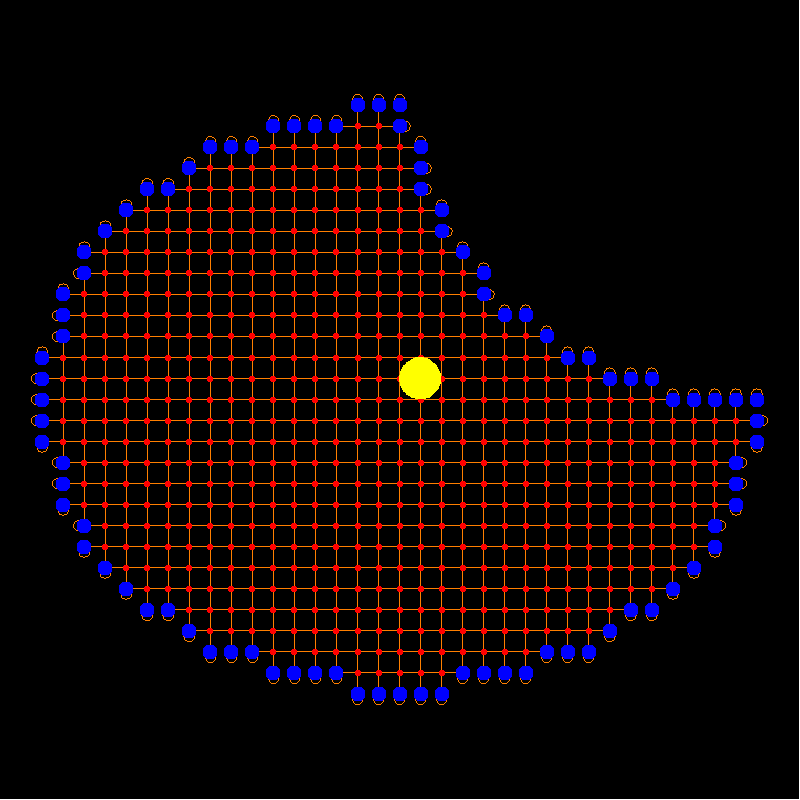M A T H 2 1 B
Mathematics Math21b Spring 2007
Linear Algebra and Differential Equations
Exhibit: diffusionDiffusion on a discrete network can be computed with linear algebra. For this illustration, the computer was iterating the adjency matrix A of the graph, computing so the exact number of paths of length up to 100 from any point to any other point.